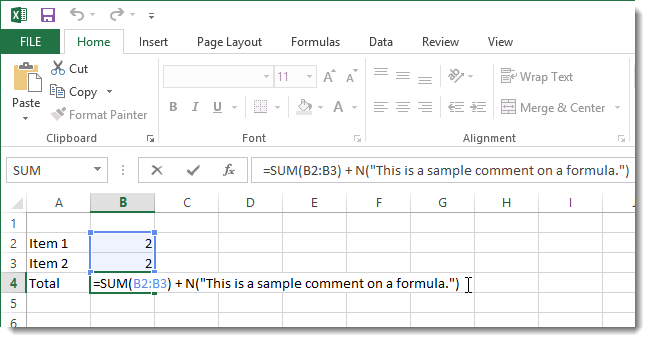# In the continuation of Excel application tutorials, here we fully teach you how to write formulas in Excel 2010-2016 software.

In fact, one of the most powerful features in Excel 2010 to 2016 software is the ability to calculate practical information using formulas.

Like a calculator, Excel can add, subtract, multiply or divide. In this lesson, we showed that we can use home resources to create a simple formula.

## Educational film

If you can learn better through video, learn formula writing in Excel from the following video:

## Mathematical operators

Excel uses standard operators on formulas, including the + sign for addition, the – sign for subtraction, the star for multiplication, / for division, and the card for views. It is better to get acquainted with how to  work with cells completely in Excel . And even before that how to set up and get started with Excel software !

### Description of Mathematical Operators

1- The sign “+”: plural

2- The sign “-“: subtraction

3- The sign “/”: division

4- The sign “*”: multiplication

5- The sign “^”: power

To write a formula in Excel, the first thing to do is put a “=” in the cell. An equal sign in Excel indicates that you intend to write the formula in the corresponding cell. In addition to the equal sign, positive and negative symbols can also be used but are not recommended. Formula writing in Excel has a structure and elements that we will mention in the following.